# introduction

A method to simulate pooled sequencing data (Pool-seq) is implemented in the R language in the package poolHelper. The aim of this package is to provide users with a tool to chose the appropriate pool size and depth of coverage when conducting experiments that require pool sequencing. This vignette serves as a introduction, explaining how the different functions of the package can be used to assess the impact of different sequencing parameters.

At the end, we also included a section with details of specific functions. At that section, users can find a detailed step-by-step description of how to simulate Pool-seq data. The various subsections describe how to simulate the total depth of coverage and then partition that coverage among different pools and individuals. There is also a subsection describing how the number of reads with the reference allele can be computed according to the genotype of a given individual.

library(poolHelper)

With the poolHelper package, users can evaluate the effect of different pool errors, pool sizes and depths of coverage on the allele frequencies. The frequencies obtained with Pool-seq are compared to the allele frequencies computed directly from genotypes.

# Basic functionality

Briefly, we use scrm to simulate a single population at equilibrium and obtain polymorphic sites for each simulated locus. Then, we compute allele frequencies by counting the total number of derived alleles per site and dividing that by the total number of gene copies. After obtaining the allele frequencies computed directly from genotypes, we simulate Pool-seq data and obtain the Pool-seq allele frequencies. Details on this procedure can be found in the last section of this vignette.

We then use the mae function from the Metrics package to compute the average absolute difference between the Pool-seq allele frequencies and the ones obtained directly from the genotypes. Mean Absolute Error (MAE) is calculated as the sum of absolute errors divided by the sample size.

# Pool-seq experimental design

As mentioned, the main goal of the package poolHelper is to provide users with a tool to aid in the experimental design of pooled sequencing. Researchers interested in Pool-seq are concerned in obtaining accurate estimates of allelic frequencies, while keeping the costs down. Thus, it is important to have an idea of how accurate the allele frequencies can be when using different pool sizes or sequencing at different mean coverage values. In the following sections we detail how the poolHelper package can help users in answering those questions.

## How many pools should I use?

One important aspect to consider is whether DNA extraction should be done using multiple batches of individuals, combining several of them into larger pools for library preparation and sequencing, or using a single batch of individuals. By using the maePool function we can check, under different conditions, what is the effect of using multiple or a single batch of individuals.

The pools input argument allows the user to simulate a single pool, by creating a list with a single integer or multiple pools, by creating a list with a vector containing various entries. The maePool function assumes that each entry of that vector is the size, in number of diploids individuals, of a given pool.

# create a list with a single pool of 100 individuals
pools <- list(100)
# compute average absolute difference between allele frequencies
onePool <- maePool(nDip = 100, nloci = 1000, pools = pools, pError = 100, sError = 0.01,
mCov = 100, vCov = 250, min.minor = 0)

# create a list with 10 pools, each with 10 individuals
pools <- list(rep(10, 10))
# compute average absolute difference between allele frequencies
tenPool <- maePool(nDip = 100, nloci = 1000, pools = pools, pError = 100, sError = 0.01,
mCov = 100, vCov = 250, min.minor = 0)

# combine both
final <- rbind(onePool, tenPool)
# convert the number of individuals in the pool to a factor
final$nPools <- as.factor(final$nPools)

library(ggplot2)
# MAE value in the y-axis and the number of individuals in the pool in the
# x-axis
ggplot(final, aes(x = nPools, y = absError)) + geom_boxplot() + theme_classic()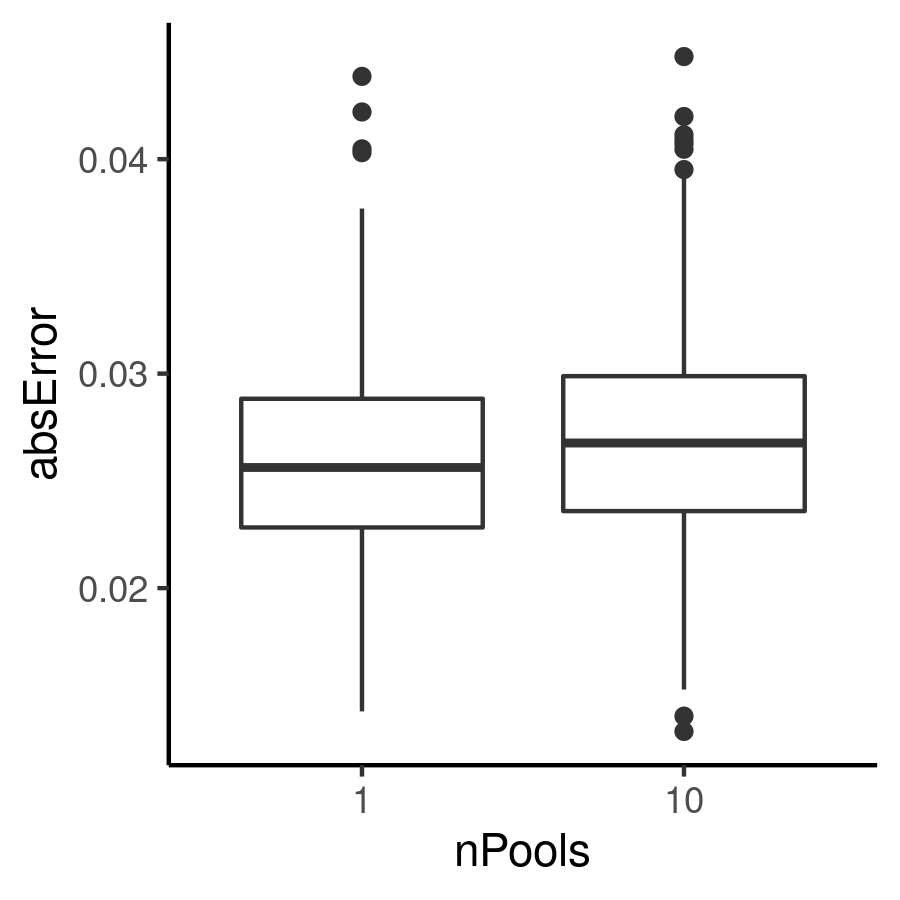In this example, we can see the effect of using a single or multiple pools when a sample of 100 individuals is sequenced at a mean coverage of 100x and for a given pool error. By varying the pError and mCov input arguments, users can evaluate the effect of using a single or multiple pools at various pool error values and at different coverages.

## What coverage should I use?

Another fundamental decision is what mean coverage should we try to obtain when sequencing a pool of individuals. By using the maeFreqs function we can look at the average absolute difference between genotype allele frequencies and Pool-seq allele frequencies obtained using different mean coverages.

# create a vector with various mean coverages
mCov <- c(20, 50, 100)
# create a vector with the variance of the coverage
vCov <- c(100, 250, 500)
# compute average absolute difference between allele frequencies
mydf <- maeFreqs(nDip = 100, nloci = 1000, pError = 100, sError = 0.01, mCov, vCov,
min.minor = 0)

# convert the mean coverage into a factor
mydf$mean <- as.factor(mydf$mean)
# boxplot the MAE value in the y-axis and the coverage in the x-axis
ggplot(mydf, aes(x = mean, y = absError)) + geom_boxplot() + theme_classic()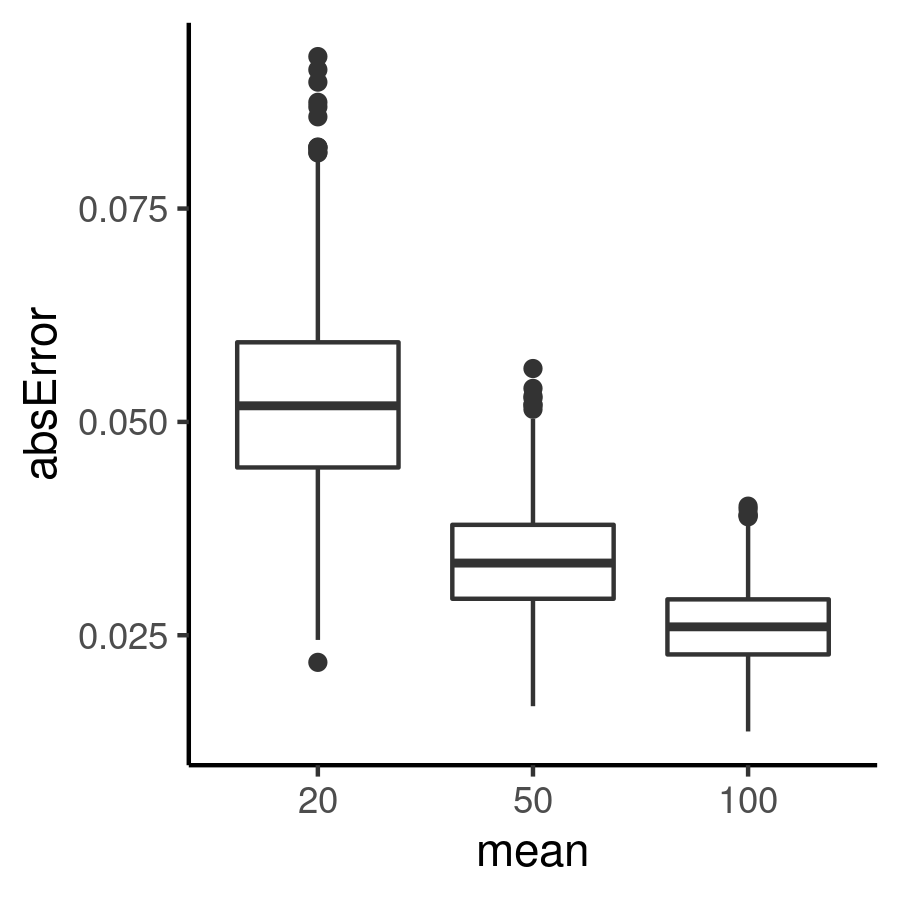Note that the mCov input argument is a vector with various mean coverage values. The maeFreqs function computes the average absolute difference for each user-defined coverage. Additionally, vCov should also be a vector, with each entry being the variance of the corresponding coverage in mCov. In this example, we can see the effect of sequencing a sample of 100 individuals at 20x, 50x or 100x mean coverage. By varying the mCov or pError input arguments, users can evaluate the impact of different mean coverages at various pool error values.

## What pool size should I use?

It is also important to define the number of individuals to sequence or, in other words, the pool size. The maeFreqs function can also be used to compute the average absolute difference between the allele frequencies computed from genotypes and Pool-seq allele frequencies obtained with different pool sizes.

# create a vector with various mean coverages
nDip <- c(10, 50, 100)

# compute average absolute difference between allele frequencies
mydf <- maeFreqs(nDip = nDip, nloci = 1000, pError = 100, sError = 0.01, mCov = 100,
vCov = 250, min.minor = 0)

# convert the number of individuals into a factor
mydf$nDip <- as.factor(mydf$nDip)
# boxplot the MAE value in the y-axis and the coverage in the x-axis
ggplot(mydf, aes(x = nDip, y = absError)) + geom_boxplot() + theme_classic()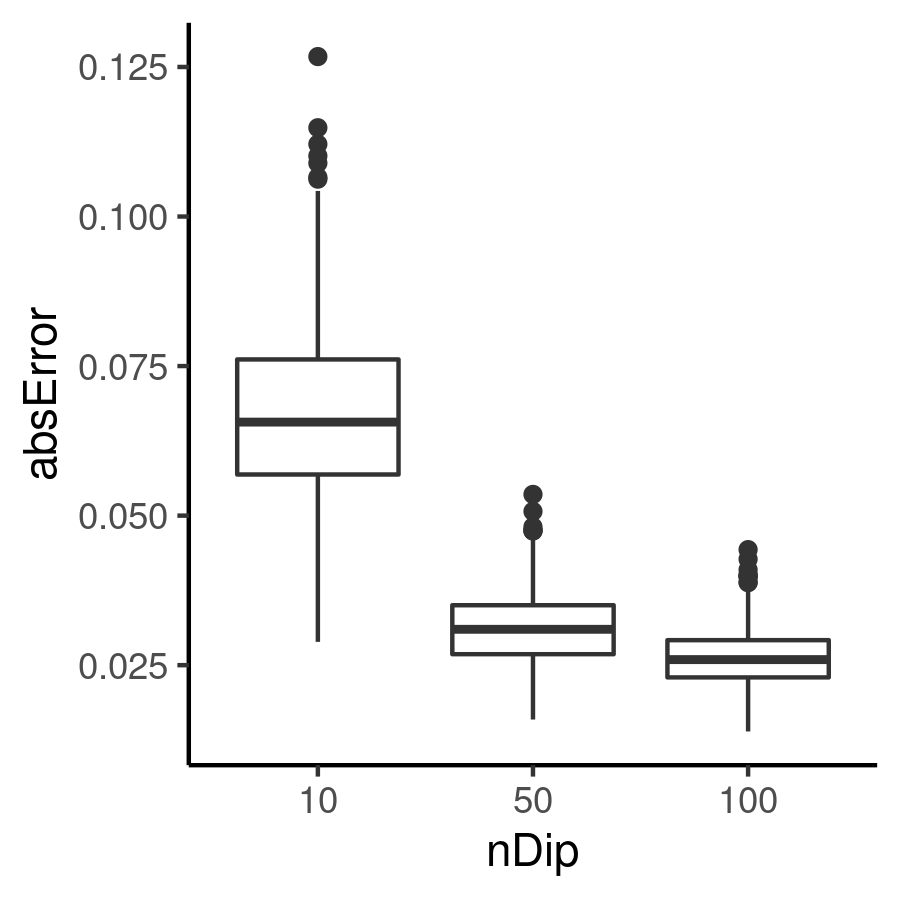As you can see, by varying the nDip input argument, we can evaluate what is the optimal pool size. In this example, we can see the effect of sequencing a sample of 10, 50 or 100 individuals at 100x coverage. For this coverage and pool error value, it is clear that doubling the pool size, from 50 to 100 individuals, does not lead to a significant decrease in the average absolute difference between allele frequencies. Note that the maeFreqs function assumes that only a single pool was used to sequence the population and so, for this example, a single pool of 10, 50 or 100 individuals was used.

## How to test different combinations?

The maeFreqs function can also be used to simultaneously test different combinations of parameters. By varying the mCov, pError and/or nDip input arguments, the impact of multiple combinations of those parameters can be quickly assessed. The maeFreqs function will simulate all possible combinations of those parameters and compute the average absolute difference between allele frequencies.

# create a vector with various mean coverages
mCov <- c(20, 50, 100)
# create a  vector with the variance of the coverage
vCov <- c(100, 250, 500)
# create a vector with various pool errors
pError <- c(5, 100, 250)

# compute average absolute difference between allele frequencies
mydf <- maeFreqs(nDip = 100, nloci = 1000, pError, sError = 0.01, mCov, vCov, min.minor = 0)

# convert the mean coverage into a factor
mydf$mean <- as.factor(mydf$mean)
# convert the pooling error to a factor
mydf$PoolError <- as.factor(mydf$PoolError)

# boxplot the MAE value in the y-axis and the pool error in the x-axis
# producing one boxplot for each of the different coverages
ggplot(mydf, aes(x = PoolError, y = absError, fill = mean)) +
geom_boxplot() + theme_classic()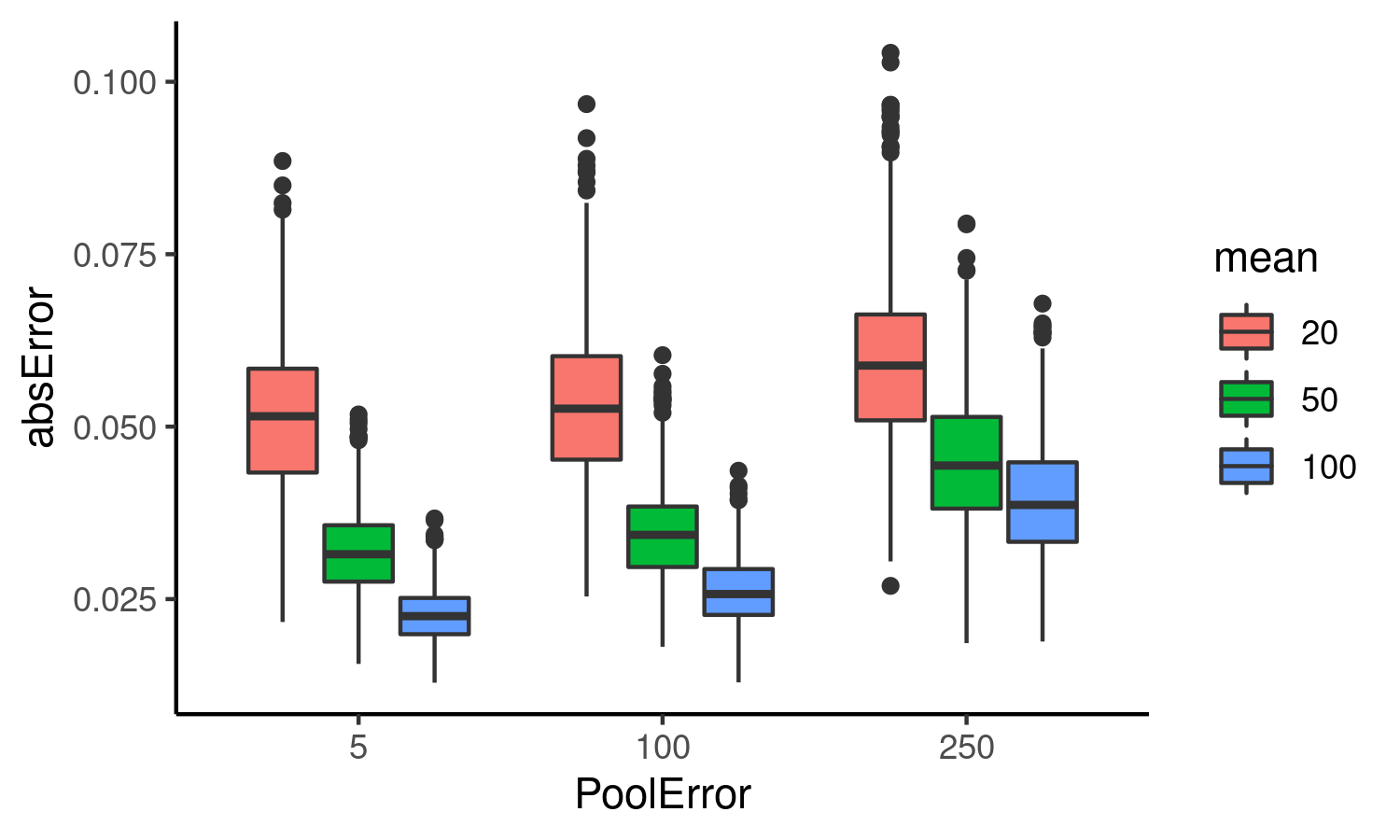In this example, the number of sampled individuals was kept constant, meaning that the population was always sequenced using a pool of 100 individuals. Those 100 individuals were sequenced at 20x, 50x or 100x mean coverage and assuming a pool error value of 5%, 100% or 250%. By selecting multiple combinations of parameters, users can select a sequencing design that minimizes the average absolute difference between allele frequencies or get an idea of how much mismatch to expect in their Pool-seq data.

# Details on specific functions

In this section and until the end of the vignette, we go over the steps required to simulate Pool-seq data and give details on some of the specific functions included in the package.

## Simulate depth of coverage

The simulateCoverage function can be used to simulate the total depth of coverage at each site. The mean and variance input arguments of the function represent, respectively the mean coverage and the variance of the coverage to simulate. nLoci represents the number of independent loci to simulate and nSNPs is the number of polymorphic sites to simulate per locus.

# simulate number of reads for one population
reads <- simulateCoverage(mean = 50, variance = 250, nSNPs = 100, nLoci = 1)
# display the structure of the reads object
#> List of 1
#>  $: int [1, 1:100] 45 25 52 24 44 30 44 50 57 51 ... As you can see, the resulting output is a list with one entry because nLoci = 1. That entry is a vector with length = 100 because that was the number of nSNPs. We can also use this function to simulate the coverage of multiple populations at the same time. To do that, the mean and variance input arguments of the function should be vectors. The function will assume that each entry of those vectors is the mean and variance of a different population. For instance, in the next example we set mcov <- c(50, 100), meaning that we wish to simulate two populations, the first with a mean coverage of 50x and the second with a mean coverage of 100x. # create a vector with the mean coverage of each population mcov <- c(50, 100) # create a vector with the variance of the coverage for each population vcov <- c(250, 500) # simulate number of reads for two populations reads <- simulateCoverage(mean = mcov, variance = vcov, nSNPs = 100, nLoci = 1) # display the structure of the reads object str(reads) #> List of 1 #>$ : int [1:2, 1:100] 48 79 19 142 41 97 42 102 34 133 ...

Now, the output of the function is slightly different. We still have a single locus (nLoci = 1) and 100 sites on that locus (nSNPs = 100) but now that one list entry is a matrix with two rows. Each row is the coverage per site for one population. Thus, the first row is the coverage for the first population of the mcov input argument and the second row is the coverage for the second population in that argument. If mcov had the mean coverage for more populations, the logic would remain the same.

The difference in the mean coverage of the two population can be quickly visualized. In the following we simulate two populations, one with 50x mean coverage and the other with 100x. We set nSNPs = 10000 and visualize the coverage distribution using a histogram.

# create a vector with the mean coverage of each population
mcov <- c(50, 100)
# create a vector with the variance of the coverage for each population
vcov <- c(250, 500)
# simulate number of reads for two populations
reads <- simulateCoverage(mean = mcov, variance = vcov, nSNPs = 10000, nLoci = 1)
# plot the coverage of the first population
hist(reads[][1,], col = rgb(0,0,1,1/4), xlim = c(0, 200), main = "", xlab = "")
# add the coverage of the second population
hist(reads[][2,], col = rgb(1,0,0,1/4), add = TRUE)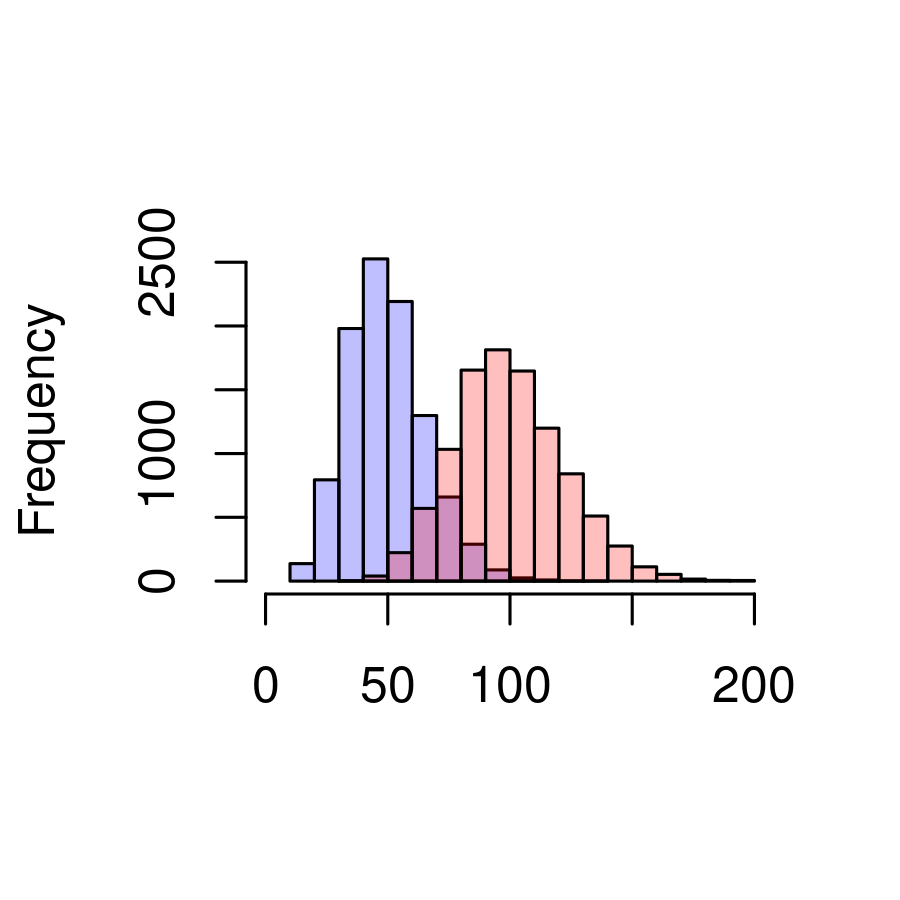The coverage distribution of the population simulated with a mean of 50x is shown in blue and the distribution of the 100x population is shown in red.

It is also possible to remove sites with low or high coverage by using the remove_by_reads function. This function will completely remove any site from the data (in this instance, the site will be removed from both populations). Sites will be removed if their coverage is below the minimum allowed or if it is above the maximum allowed. In the next bit, we use the reads simulated before and remove all sites with a coverage below 25x and above 150x.

# check the minimum and maximum coverage before removal
# remove sites with coverage below 25x and above 150x
# display the structure of the reads object after removal
#> List of 1
#>  \$ : int [1:2, 1:9478] 70 129 57 123 33 67 44 103 56 91 ...
# check the minimum and maximum coverage after removal
#>   25 150

Accordingly, the minimum simulated coverage before running the remove_by_reads function was 11 and the maximum was 200 but after removal of sites with a coverage below 25x and above 150x, the minimum and maximum coverage are, obviously, 25 and 150 respectively. It is also clear that we no longer have nSNPs = 10000 in the data.

## Reads contributed by each pool

It is also possible to simulate the contribution of each pool, assuming that a single population was sequenced using multiple pools. Before computing the actual number of reads contributed by each pool, we first need to simulate the proportion of contribution.

To do this, we use the poolProbs function. The nPools input argument of this function should represent the number of pools used to sequence the population, while the vector_np contains the number of individuals per pool. Thus, in the following example vector_np = c(10, 10, 10, 10) means that four pools were used to sequence the population, each comprised of 10 individuals. The pError input argument defines the degree of pooling error. Briefly, this pooling error controls the dispersion of the pool contribution, centered around the expected value. Higher values of pError lead to a higher dispersion and thus, the contributions will vary more between pools. In other words, with higher values of pError some pools will contribute a lot of reads and others will not contribute much.

In the next chunk, we see the difference in proportion of contribution when 4 pools of 10 individuals were used to sequence a single population and the pooling error is either low (pError = 5) or high (pError = 250). We can also assess the impact of different pool sizes by including one pool with 100 individuals instead of only 10.

# four pools with low sequencing error
poolProbs(nPools = 4, vector_np = c(10, 10, 10, 10), nSNPs = 6, pError = 5)
#>           [,1]      [,2]      [,3]      [,4]      [,5]      [,6]
#> [1,] 0.2531750 0.2521152 0.2502917 0.2505605 0.2519052 0.2530544
#> [2,] 0.2550141 0.2485521 0.2507695 0.2464415 0.2515553 0.2432920
#> [3,] 0.2437321 0.2516073 0.2480703 0.2477004 0.2445733 0.2482569
#> [4,] 0.2480788 0.2477254 0.2508684 0.2552976 0.2519663 0.2553967
# four pools with high sequencing error
poolProbs(nPools = 4, vector_np = c(10, 10, 10, 10), nSNPs = 6, pError = 250)
#>            [,1]       [,2]       [,3]        [,4]       [,5]       [,6]
#> [1,] 0.35203040 0.11721727 0.24999286 0.053257800 0.17053795 0.21050741
#> [2,] 0.34600661 0.47759585 0.09486927 0.008174306 0.46725116 0.57239464
#> [3,] 0.04304608 0.03595768 0.14154901 0.392971715 0.34067521 0.03439114
#> [4,] 0.25891690 0.36922921 0.51358886 0.545596180 0.02153568 0.18270680
# four pools but one is much larger
poolProbs(nPools = 4, vector_np = c(10, 100, 10, 10), nSNPs = 6, pError = 5)
#>            [,1]       [,2]       [,3]       [,4]       [,5]       [,6]
#> [1,] 0.07641779 0.07765904 0.07636717 0.07708453 0.07698771 0.07521794
#> [2,] 0.77220887 0.77090425 0.76835073 0.76929348 0.77021613 0.77247130
#> [3,] 0.07624709 0.07776729 0.07762311 0.07649797 0.07681877 0.07546535
#> [4,] 0.07512626 0.07366943 0.07765898 0.07712402 0.07597739 0.07684541

The output of the poolProbs function is a matrix with the proportion of contribution for each pool. Each row of the matrix corresponds to a different pool and each column is a different site. You can see that in the first example, the proportion of contribution is roughly the same for all pools. The next example is similar but with pError = 250. With this higher pool error, it is clear that some pools have a higher proportion of contribution and others have a smaller. Thus, with higher pool errors, the proportion of contribution is no longer the same for all pools.

This also happens when pool error is low but one of the pools is much larger. In the last example, the second pool has 100 individuals, while the other pools only have 10. In this instance, it is clear the the proportion of contribution of the larger pool is always higher.

After computing the proportion of contribution of each pool, this can be used to simulate the actual number of reads contributed by each pool. To do this, we use the pReads function.

This functions requires as input argument the total number of pools used to sequence the population (nPools), a vector with the total coverage per site and the probabilities of contribution computed with the poolProbs function (probs). In the next chunk, we simulate coverage for 10 SNPs of a single population, compute the probability of contribution for 4 pools used to sequence that population and then simulate the actual number of reads per pool.

# simulate total coverage per site
reads <- unlist(simulateCoverage(mean = 100, variance = 250, nSNPs = 10, nLoci = 1))
# compute the proportion of contribution of each pool
probs <- poolProbs(nPools = 4, vector_np = rep(10, 4), nSNPs = 10, pError = 5)
# simulate the contribution in actual read numbers
# output the number of reads per pool and per site
#>      [,1] [,2] [,3] [,4] [,5] [,6] [,7] [,8] [,9] [,10]
#> [1,]   22   23   18   18   22   33   34   28   16    18
#> [2,]   22   31   30   23   27   24   36   28   28    21
#> [3,]   31   29   27   25   23   19   36   23   26    27
#> [4,]   21   23   32   23   33   25   26   28   21    23

It is clear that, when pool error is quite low (pError = 5 in the previous chunk), the number of reads contributed by each pool is quite similar. Thus, the total coverage of any given site is well distributed among all pools. On the other hand, if pool error is high (pError = 250 in the next chunk).

# simulate total coverage per site
reads <- unlist(simulateCoverage(mean = 100, variance = 250, nSNPs = 10, nLoci = 1))
# compute the proportion of contribution of each pool
probs <- poolProbs(nPools = 4, vector_np = rep(10, 4), nSNPs = 10, pError = 250)
# simulate the contribution in actual read numbers
# output the number of reads per pool and per site
#>      [,1] [,2] [,3] [,4] [,5] [,6] [,7] [,8] [,9] [,10]
#> [1,]   32   31   57   12   62   48   45   21   30    12
#> [2,]   11    4   14   60    4   11    1   27   18    20
#> [3,]   21    6   24    0    5    3    4   10   35    31
#> [4,]   43   34   17    5   32   37   46   30    4    27

Then the contributions are more uneven. In this instance, there are some sites where one or two pools contribute most of the reads while the remaining pools have few or even zero reads. Thus, the total coverage is not very well distributed among all pools when pool error is higher.

The difference between low or high pool errors can be (roughly) inspected with a histogram. In the next chunk we simulate the total coverage and then use the same coverage to compute the contribution of each pool, using either a low or a high pool error. We then plot the distribution of the number of reads contributed by each pool.

# simulate total coverage per site
reads <- simulateCoverage(mean = 100, variance = 250, nSNPs = 10000, nLoci = 1)
# unlist to create a vector with the coverage

# compute the proportion of contribution of each pool
probs <- poolProbs(nPools = 4, vector_np = rep(10, 4), nSNPs = 10000, pError = 5)
# simulate the contribution in actual read numbers

# compute the proportion of contribution of each pool
probs <- poolProbs(nPools = 4, vector_np = rep(10, 4), nSNPs = 10000, pError = 250)
# simulate the contribution in actual read numbers

# create the plot of the contribution with low pool error
h1 <- hist(unlist(low.pReads), plot = FALSE)
# create the plot of the contribution with high pool error
h2 <- hist(unlist(high.pReads), plot = FALSE)
# get the maximum x-value from the two plots
xmax <- max(h1[["breaks"]], h2[["breaks"]])
# and the maximum y-value
ymax <- max(h1[["counts"]], h2[["counts"]])
# set the color for the contribution computed with low pool error
col1 <- rgb(0, 0, 1, 1/4)
# set the color for the contribution computed with high pool error
col2 <- rgb(1, 0, 0, 1/4)

# plot the contribution computed with low pool error
plot(h1, col = col1, xlim = c(0, xmax), ylim = c(0, ymax), main = "", xlab = "")
# add the plot of the contribution computed with high pool error
plot(h2, col = col2, add = TRUE)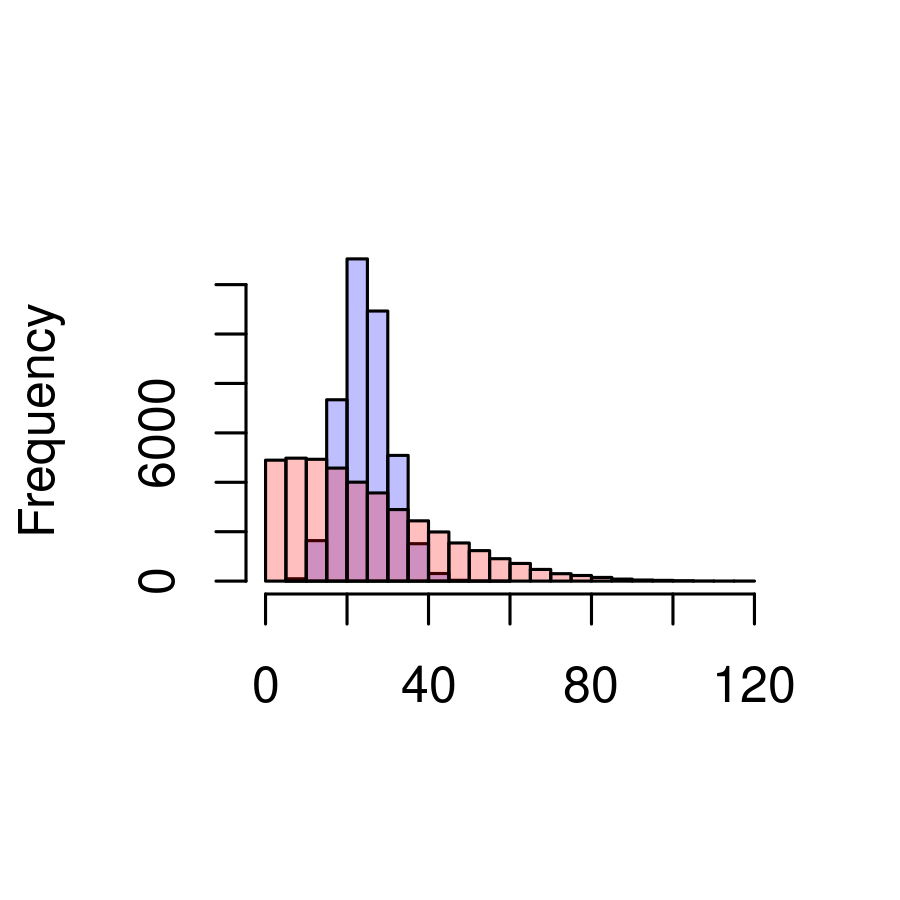The distribution of the contribution computed with a low pool error is shown in blue and the distribution computed with a high pool error in red. It is clear that high pool errors lead to more variation in the contribution of each pool towards the total coverage of the population. In particular, the number of pools that contribute zero (or close to zero) reads increases when the pool error is high.

## Reads contributed by each individual

After computing the number of reads contributed by each pool, the next step involves simulating the number of reads contributed by each individual inside their pool. For instance, if a pool of 10 individuals was used to sequence a population, how many reads were contributed by each of those 10 individuals?

As for the pools, the first step requires computing the probability of contribution of each individual. This can be done with the indProbs function. This np input argument of this function corresponds to the total number of individuals in the pool, while the nSNPs is the number of sites to simulate. As before, the pError represents the degree of pooling error and higher values of pError mean that some individuals will contribute more reads than others.

In the next chunk, we examine the probability of contribution of 10 individuals, sequenced at 6 sites, when pooling error is quite low.

# compute the probability of contribution of each individual
indProbs(np = 10, nSNPs = 6, pError = 5)
#>             [,1]       [,2]       [,3]       [,4]       [,5]       [,6]
#>  [1,] 0.09942878 0.10489291 0.09390599 0.10272734 0.10140531 0.10624918
#>  [2,] 0.09478936 0.10611204 0.10619381 0.10866073 0.09437200 0.09267993
#>  [3,] 0.09898130 0.10051992 0.10549628 0.10395796 0.10420805 0.09716645
#>  [4,] 0.09862546 0.10139381 0.09286409 0.09395518 0.09944341 0.10137621
#>  [5,] 0.09696531 0.09223213 0.10354205 0.10787825 0.10273086 0.10699659
#>  [6,] 0.10299649 0.09590832 0.11223930 0.09631223 0.09683748 0.09736608
#>  [7,] 0.10036560 0.09992958 0.10456514 0.09737974 0.10254431 0.10042422
#>  [8,] 0.10712639 0.09488659 0.09302650 0.09069288 0.09947049 0.09374426
#>  [9,] 0.10138287 0.10109722 0.10139368 0.09787590 0.09709237 0.10920492
#> [10,] 0.09933843 0.10302748 0.08677316 0.10055979 0.10189573 0.09479215

In this example, the probability of contribution is very similar across individuals. In fact, the probability is around 0.1 for each individual, meaning that, in a situation with low pooling error, all individuals should contribute equally. If we simulate the same conditions, but increasing the pooling error (pError = 150) we should see a different result. Note that we use the round function so that the the output is not printed in scientific notation. This is just to make it easier to visualize the differences.

# compute the probability of contribution of each individual
round(indProbs(np = 10, nSNPs = 5, pError = 150), digits = 5)
#>          [,1]    [,2]    [,3]    [,4]    [,5]
#>  [1,] 0.96909 0.00190 0.13819 0.02656 0.00027
#>  [2,] 0.00203 0.08211 0.00260 0.22690 0.12091
#>  [3,] 0.00019 0.47912 0.07474 0.00092 0.00567
#>  [4,] 0.00072 0.00111 0.06580 0.00013 0.24133
#>  [5,] 0.00168 0.14395 0.16019 0.01916 0.16992
#>  [6,] 0.01196 0.15634 0.00005 0.09524 0.00000
#>  [7,] 0.01421 0.12506 0.00139 0.52440 0.20379
#>  [8,] 0.00003 0.00652 0.04360 0.05050 0.01049
#>  [9,] 0.00000 0.00374 0.51317 0.01651 0.00327
#> [10,] 0.00010 0.00013 0.00026 0.03969 0.24433

With this higher pooling error, it is evident that the probability of contribution is not the same across all individuals. Some individuals have a much higher probability of contribution while others have a probability of contribution very close to zero.

The probabilities of contribution of each individual can then be used to simulate the total number of reads contributed by each individual, using the indReads function. This function requires as input argument the total number of individuals sequenced in that pool (np), a vector with the total coverage of that particular pool per site and probabilities of contribution computed with the indProbs function (probs).

In the next chunk, we start by simulating the total coverage per site. This total coverage is then partitioned among the different pools to obtain the total coverage per pool. Finally, we simulate the contribution of the 10 individuals sequenced at one of the pools towards the total coverage of that pool. All these steps are done assuming a low pooling error.

# simulate total coverage per site
reads <- unlist(simulateCoverage(mean = 100, variance = 250, nSNPs = 12, nLoci = 1))
# compute the proportion of contribution of each pool
probs <- poolProbs(nPools = 4, vector_np = rep(10, 4), nSNPs = 12, pError = 5)
# simulate the contribution in actual read numbers
# compute the proportion of contribution of each pool
probs <- indProbs(np = 10, nSNPs = 12, pError = 5)
# simulate the contribution in actual read numbers of each individual
#>       [,1] [,2] [,3] [,4] [,5] [,6] [,7] [,8] [,9] [,10] [,11] [,12]
#>  [1,]    2    3    4    3    3    0    1    1    5     4     4     0
#>  [2,]    0    4    1    3    1    4    0    5    2     1     3     6
#>  [3,]    4    3    0    1    4    0    0    1    3     3     3     6
#>  [4,]    2    1    2    1    4    5    1    1    2     4     1     3
#>  [5,]    0    2    2    4    5    0    4    3    1     5     0     1
#>  [6,]    2    0    7    1    1    3    4    3    2     3     2     2
#>  [7,]    1    2    2    3    2    1    3    2    5     1     5     4
#>  [8,]    3    1    2    4    2    1    1    5    1     2     2     5
#>  [9,]    8    4    4    3    0    0    5    3    6     2     6     4
#> [10,]    3    7    2    3    2    1    2    5    5     0     3     2

It is clear that, when pool error is low (pError = 5), each individual contributes roughly the same number of reads towards the total coverage of the pool. Thus, the overall dispersion is quite low. If we repeat the same steps, changing only the pooling error to a much higher value:

# simulate total coverage per site
reads <- unlist(simulateCoverage(mean = 100, variance = 250, nSNPs = 12, nLoci = 1))
# compute the proportion of contribution of each pool
probs <- poolProbs(nPools = 4, vector_np = rep(10, 4), nSNPs = 12, pError = 150)
# simulate the contribution in actual read numbers
# compute the proportion of contribution of each pool
probs <- indProbs(np = 10, nSNPs = 12, pError = 150)
# simulate the contribution in actual read numbers of each individual
#>       [,1] [,2] [,3] [,4] [,5] [,6] [,7] [,8] [,9] [,10] [,11] [,12]
#>  [1,]    0    2    0    0    0    0    3    8    0     0     2     4
#>  [2,]    1    6    0    1    0    2    0    0    9     0    11     0
#>  [3,]    1    0    0    5    0    8    0    5    0    10     0     2
#>  [4,]    0    0   23   13    0    0    0    2    0     0     1     4
#>  [5,]    1    1   11    0    0    0    1    3    0     1     3     1
#>  [6,]    2    0    0    0    2   23    4    0    7     1     0     0
#>  [7,]    0   11    0    0    0    0    2    2    0     1     0     1
#>  [8,]    2    0    0    0    8    0    6    0    0     0     6     0
#>  [9,]    1    0    1    0    9    0    6    7    5     5     3     0
#> [10,]   14    0   28    0    0    8    0    0    2     2     9     0

We see that in this instance, there is much more dispersion and the individuals do not contribute the same number of reads. While some individuals do not contribute a single reads towards the total pool coverage, others contribute too many.

## Number of reads with the reference allele

Following the computation of the number of reads contributed by each individual, we should simulate how many of those reads have the reference allele versus how many have the alternative allele. For a single population this can be done using the computeReference function.

This function requires as input argument the individual contribution i.e. the number of reads that each individual contributes and the sequencing error - error. The sequencing error is defined as a error rate - the higher the error, the more likely it is for an individual that is homozygous for the reference allele (coded as 0 in the genotypes matrix) to contribute reads with the alternative allele. Note that this function also requires as input argument the genotypes of the individuals. Given that we did not simulate genotypes in this vignette, we are going to create a matrix of genotypes where half the individuals are homozygous for the reference allele and the other half is homozygous for the alternative allele (coded as 2 in the genotypes matrix).

In the next chunk we go over all the previous steps, simulating the total coverage for one population, then partitioning that over all pools and computing the contribution of each individual in one of those pools. At the end, we simulate how many of those individually contributed reads have the reference allele.

# simulate total coverage per site
reads <- unlist(simulateCoverage(mean = 100, variance = 250, nSNPs = 12, nLoci = 1))
# compute the proportion of contribution of each pool
probs <- poolProbs(nPools = 4, vector_np = rep(10, 4), nSNPs = 12, pError = 5)
# simulate the contribution in actual read numbers
# compute the proportion of contribution of each pool
probs <- indProbs(np = 10, nSNPs = 12, pError = 5)
# simulate the contribution in actual read numbers of each individual
# create fake genotypes - half the matrix is 0 and the other half is 2
geno <- rbind(matrix(0, nrow = 5, ncol = 12), matrix(2, nrow = 5, ncol = 12))
# simulate the number of reference reads
computeReference(genotypes = geno, indContribution = iReads, error = 0.001)
#>       [,1] [,2] [,3] [,4] [,5] [,6] [,7] [,8] [,9] [,10] [,11] [,12]
#>  [1,]    1    2    1    0    1    2    4    3    2     1     5     2
#>  [2,]    3    0    1    2    7    5    2    6    3     2     2     0
#>  [3,]    5    1    4    1    1    4    2    1    2     1     1     3
#>  [4,]    2    4    3    1    3    2    2    1    6     1     2     0
#>  [5,]    0    3    2    1    0    4    1    2    4     2     1     5
#>  [6,]    0    0    0    0    0    0    0    0    0     0     0     0
#>  [7,]    0    0    0    0    0    0    0    0    0     0     0     0
#>  [8,]    0    0    0    0    0    0    0    0    0     0     0     0
#>  [9,]    0    0    0    0    0    0    0    0    0     0     0     0
#> [10,]    0    0    0    0    0    0    0    0    0     0     0     0

There is a clear division between the number of reads with the reference allele for the first 5 individuals (coded as 0 in the genotypes matrix) and the remaining 5 individuals (coded as 2 in the genotypes matrix). This is expected because the error was small. If we increased the error, then we would expect to see some reference allele reads contributed by individuals that are homozygous for the other allele.